Home > ACC7 > Chapter cc27 > Lesson cc27.1.6 > Problem7-75

7-75.
1. Ages of golfers participating in a golf tournament were 44, 48, 40, 25, 28, 37, 29, 34, 45, 51, 43, 35, 38, 57, 50, 35, 47, 30, 61, 43, 44, 60, 46, 43, 33, 45, 42, 34, 32, and 74. Homework Help ✎

1. Create a stem-and-leaf plot for this data.

2. Use the stem-and-leaf plot to create a histogram.

3. Describe the shape and spread of the data. Are there any apparent outliers?

4. Use the appropriate measure of center to describe the “typical” age of golfers at the tournament.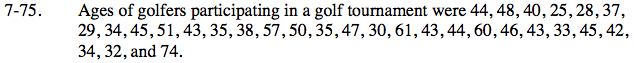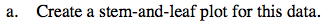Refer to the Math Notes box in Lesson 7.1.1 to review stem-and-leaf plots and histograms.

The “stem” part of the graph represents all of the digits in a number except the last one. The “leaf” part of the graph represents the last digit of each of the numbers.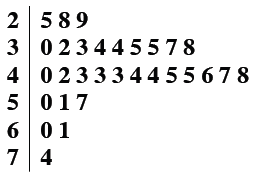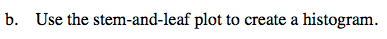In histograms, the intervals for the data are shown on the horizontal axis and the frequency (number of pieces of data in each interval) is represented by the height of a bar above the interval.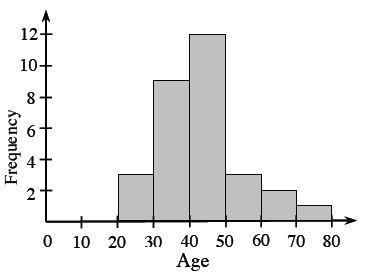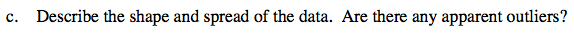Is the graph symmetric? How many peaks does it have?
Are any of the data points significantly large or small compared to the rest of the data?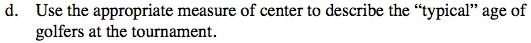What is the value of the median? What is the value of the mean? Considering the outlier, which
value do you think is better suited to describe the typical age of the golfers at the tournament?